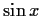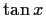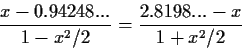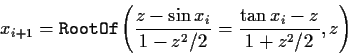Next: Transcendental Iterators. Up: Building additional iterators Previous: Polynomial iterators.

#### Substitute all non-polynomials.

Another possible way of constructing an iterator is to inspect the equation top-down and force the evaluation of any subexpression which is a special function, a power to a non-integer exponent, or anything else that is not algebraic. If after this is done, we are left with an expression containing x, this must be a rational polynomial expression. The solution of this is equivalent to the solution of a polynomial and we can proceed as with the previous iterators. For examplefor x0=1.23 would be handled by evaluating theand, obtainingwhich has the roots -3.3331..., 1.2024.... From these possible values for x1 we select, as before, the closest to x0, x1=1.2024.... More formally, using Maple's RootOf notation, the iterator becomesTable 3: Rational polynomial iterators, obtained by solving all the transcendental subexpressions first
 66644 random equations producing 14398 iterators method failures converged to a root 11.64% converged to a non-root .39% failed 88.36% diverged 6.22% average time .672 outside domain 3.51% time per root 5.768 too many iterations 1.15% iterator fails 0.00% iterators per equation .216 no iterators 88.73%

Table 3 shows the simulation results for this heuristic.Next: Transcendental Iterators. Up: Building additional iterators Previous: Polynomial iterators.
Gaston Gonnet
1998-07-08